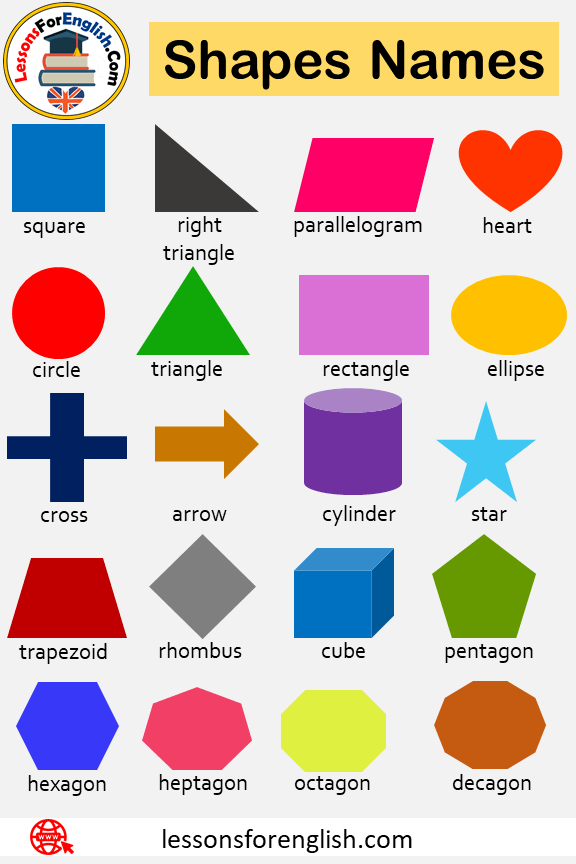# Geometric Shapes Names, Geometric Figures and Pictures

Geometric Shapes Names, Geometric Figures and Pictures#### Geometric Shapes

There are 6 geometric shapes in mathematics. Each geometric shape has its own rules and angles. Today we will tell you about these geometric shapes and their properties.

#### What are these six basic geometric shapes?

The circle is one of them. Circles are made by calculating their circumferences with a line drawn from their center to the edge. It must be the same distance from each point of the center circle.

Triangle: Triangles can be drawn in different types but always have 3 corners and sides. The sum of the angles of the triangles must always be equal to 180 degrees. The sides of the triangles are not always equal, and the triangles are divided into three in terms of sides.

Equilateral Triangle: In this type of triangle, all sides of the triangle are equal to each other. Each angle in this triangle must be 60 degrees.

Isosceles Triangle: In this type of triangle, only two sides are equal in length. The third side is of a different length.

Scalene Triangle: In this type of triangle, no sides are of equal length.

Triangles are also divided into three according to their angles.

Right triangle: The greatest angle in this triangle is a right angle. The right angle is 90 degrees.

Acute Triangle: This triangle is the largest of the three angles. Narrow angles are less than 90 degrees.

Obtuse Triangle: The biggest angle of this triangle is called the obtuse angle. Wide angles are greater than 90 degrees, ie perpendicular.

The Rectangle: A rectangle is a geometric shape in which the lengths of opposite sides are equal to each other. The angles inside are always 90 degrees and equal to each other.

The Rhombus: It is created with 4 straight lines, but not every angle is 90 degrees. The lengths of the 4 sides are equal to each other.

The Square: The four sides are equal and each angle is 90 degrees. It is similar to a rhombus in that all sides are equal to each other. Regardless of their size, all squares are parallel to each other.

The Trapezoid: The trapezoid shape has 4 sides, but as the name suggests, not all sides are equal. Only 2 opposite sides are parallel to each other.

Parallelogram: Parallelogram means that opposite sides are parallel to each other. Some parallelograms also have symmetry lines.

Kite: It has two equal sides. These edges are adjacent to each other.

Trapezium: This shape, called Trapezoid in the UK, is a quadrilateral with no sides equal or parallel.

Geometric shapes such as Pentagon, Hexagon, Heptagon, Octagon, Nonagon, Decagon also have a certain order. Apart from these, geometric shapes are divided into two as concave and convex.

Geometric shapes do not end with these. But these are basic geometric shapes that have rules and what we call smooth. The interior angles, side lengths, and parallelisms of all of them vary.